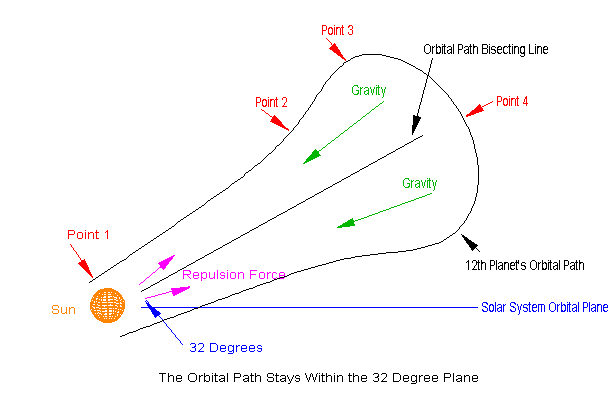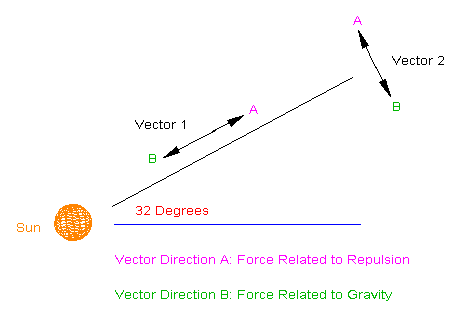Forces Acting on the 12th Planet from Point A to EA Breakdown of Forces Applied at Points 1 to 4  will be Represented by Vectors:Point 1: As the 12th planet pierces the elliptical plane and passes the Sun several forces come into play. Its approach velocity allows closer pass to the Sun inside the point of repulsion (the point where the force gravity and repulsion are equal keeping interacting masses apart). There is gravity pulling the 12th planet towards the Sun, the repulsion force pushing outwards and to a very small degree, the Dark One pulling from the rear. The repulsion force greater than the attractive force gravity within the orbit of Saturn pushes the 12th planet out of the solar system. Velocities in vectors 1 & 2 are pointed to A both at their maximums. This forms a smooth line slightly outwards from the orbital bisect line for a uniform segment of T (Time).

Point 2: In this sector of the orbital path, velocity decreases in the direction A at a greater rate in vector 1 than vector 2 due to the gravity sources of the Sun and dark One and the orbit takes on a parabolic outward curve during the same interval T.

Point 3: At this point the force of gravity as eroded all velocity due to the repulsion force perpendicular to the orbital bisecting line. In vector 2 all forces are neutral with no direction. In vector 1, velocity has slow significantly. As the 12th planet proceeds past this point, gravity bends the orbit inward and vector 2 takes on a new direction towards B.

Point 4: Here at the 12th planet’s maximum distance from the Sun, velocity in vector 1 has reached zero as gravity from both stellar objects halts forward progress. Gravity only provides movement in the direction B along vector 2 as momentum carries it pass the orbital bisecting line. It is gravity again that stops forward progress in vector 2 and the inbound path mirrors the outbound.